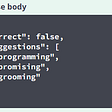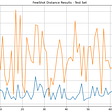# LETS UNDERSTAND THE DATASET…..

• 150 samples
• 3 labels: species of Iris (Iris setosa, Iris virginica and Iris versicolor)
• 4 features: Sepal length,Sepal width,Petal length,Petal Width in cm
`from  sklearn import  datasetsiris=datasets.load_iris()`

## Assign the data and target to separate variables.

`x=iris.datay=iris.target`

# Splitting the dataset;

`from sklearn.model_selection import train_test_splitx_train,x_test,y_train,y_test=train_test_split(x,y,test_size=.5)`

# Build the model

`from sklearn import treeclassifier=tree.DecisionTreeClassifier()`
`from sklearn import neighborsclassifier=neighbors.KNeighborsClassifier()`

# Train the Model.

`classifier.fit(x_train,y_train)`

# Make predictions:

`predictions=classifier.predict(x_test)`
`from sklearn.metrics import accuracy_scoreprint(accuracy_score(y_test,predictions))`

# Logistic Regression

`from sklearn.linear_model import LogisticRegressionfrom sklearn import metrics`
`logreg = LogisticRegression()logreg.fit(X_train, y_train)`
`y_pred = logreg.predict(X_test)print('Accuracy of logistic regression classifier on test set: {:.2f}'.format(logreg.score(X_test, y_test)))`

# Classification Report

`from sklearn.metrics import classification_reportprint(classification_report(y_test, y_pred))`

# Perceptron

`pn = Perceptron(tol=1e-3, random_state=0)pn.fit(X_train, y_train)`
`pn.score(X_train,y_train)`
`pn.score(X_test,y_test)`

# Neural Network

`from keras.models import Sequentialfrom keras.layers import Densefrom keras.utils import to_categorical`
`x_train = np.array(x_train)model = Sequential()model.add(Dense(4, input_dim=4 ,activation='relu'))model.add(Dense(7, activation='relu'))model.add(Dense(3, activation='softmax'))model.compile(loss='binary_crossentropy', optimizer='adam', metrics=['accuracy'])`
`y_test_cat=to_categorical(y_test)y_train_cat=to_categorical(y_train)model.fit(x_train, y_train_cat, epochs=149, batch_size=4)`
`_, accuracy = model.evaluate(X_train, y_train_cat)print('Accuracy: %.2f' % (accuracy*100))`
`_, accuracy = model.evaluate(X_test, y_test_cat)print('Accuracy: %.2f' % (accuracy*100))`

# Random Forest

`from sklearn.ensemble import RandomForestClassifierclassifier = RandomForestClassifier(n_estimators = 900, criterion = 'gini', random_state = 0)classifier.fit(X_train, y_train)y_pred = classifier.predict(X_test)`
`print(classifier.score(X_test, y_test)`

# SUMMARY

--

--

--

## More from Sriraag Av

Love podcasts or audiobooks? Learn on the go with our new app.

## Mercari Price Suggestion Challenge## LAMBADA Method: How to use Data Augmentation in NLU?## ML CheatSheets: Activation Functions## NLP API: Find Spelling Corrections for a Word in Go## ARTIFICIAL NEURAL NETWORK## How Deep Learning can boost Contextual Advertising Capabilities## Build a Linear Support Vector Machine Model From Scratch with Python## Metric Learning for Steel Surface Classification — Part 2## Classification Performance: Confusion Matrices